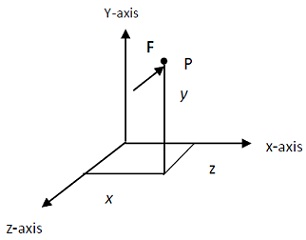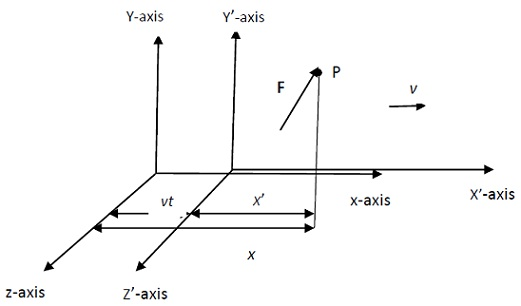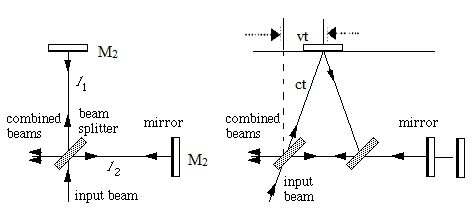#### Galilean Transformation, Physics tutorial

Introduction:

Theory of relativity is the study of relationships between observations made in different frames of reference that are in relative motion to each other. Mathematical basis of comparing two descriptions is known as transformation.

Frames of Reference:

Every physical event takes place somewhere in space (a portion of physical universe) in the definite time interval. In three-dimensional Euclidean space it is suitable to specify position of such an event using frame of reference that includes a three-dimensional rectangular coordinate system. Position of point in space in Cartesian coordinate system is defined by ordered set, (x, y, z) known as Cartesian coordinates of point. This, of course, is not the only of coordinate system.Consider the particle of mass m at a point P applied on by the force F at an instant of time t as shown in figure coordinates in space (spatial coordinates) of event as estimated by the observer at origin of the coordinate system are (x, y, z, t). Space and time are intricately intertwined. This is true as every event in nature has the definite location (space) and duration (time). As such, we speak of space-time rather than space and time separately. So, set of four numbers (x, y, z, t) tells us that at time t, coordinates of particle at point P are x, y, z.

Now, we can write Newton's second law of motion for this event in terms of components FZ, and of force F as

Fx = md2x/dt2

Fy = md2y/dt2

FZ = md2z/dt2

These equations are valid only if frame of reference explained by coordinates x, y, z is inertial. The frame of reference is said to be inertial if the object in it, which is not under influence of the force, will remain at rest if it was originally at rest or continue in its motion with constant velocity, if it was originally in motion.

Galilean Transformation:

Consider two observers O and O' at origins of frames of reference S and S' respectively, that are in relative motion at constant velocity v. Frames of reference that are in translational motion at constant velocity relative to each other are known as inertial frames of reference. Assume also that O and O' origins and axes of the coordinates of these frames are coincident at initial time t = t' = 0.Two observers are prepared with measuring instruments to find out coordinates of event at P. Measurements made in frame are related to those made in S' by Galilean transformation as follows:

x' = x - vt

y' = y

z' = z

t' = t

Let us write, equations of Newton's second law of motion for this event using such transformations for each of S and S' frames. x, y, and z components of force F which applies on particle at P for S frame.

To obtain corresponding equations in frame, first differentiate twice to get components of acceleration as follows:

dx'/dt' = dx/dt - v and d2x'/dt2 = d2x/dt2

Therefore, we can replace t by t' in above equation and write

d2x'/dt'2 = d2x/dt2

Thus, components of force in S' frame can be written in terms of components of acceleration as follows:

Fx' = md2x'/dt'2, Fy' = md2y'/dt'2, Fz' = md2z'/dt'2

Equations are invariant under Galilean transformation. Newton's laws comprise main platform on which the motions of objects are examined in classical physics and administer behavior of mechanical systems. It follows that laws which administer behavior of mechanical systems will forever have same form in all inertial frames of reference. As the example of this generalization, let two laboratory experiments performed under same conditions to find out gravitational constant g.

Non- Invariance of Electromagnetic Phenomena under Galilean Transformation:

Behavior of the electromagnetic phenomenon can be explained in terms of Maxwell's equations. It is from equations that differential equation of electromagnetic wave in free space is attained. Maxwell's equations state spatial variation with respect to time of time-dependent electric field intensity E and magnetic induction B. Here the equation of electromagnetic wave in free space is written in terms of coordinates of electric field and then try to verify invariance or otherwise under Galilean transformation.

The equation is

2E = ε0μ0∂2E/∂t2

Where ε0 and μ0 are permittivity and permeability of free space respectively. In Cartesian coordinate system electric field vector E can be written in terms of its components as

E = exEx + eyEy + ezEz, where ex, ey and ez are unit vectors in x-, y-, and z-directions respectively. Equation then separates in three equations obeyed by each of components of E. Therefore, for x - component, write equation as:

2Ex/∂x2 + ∂2Ex/∂y2 + ∂2Ex/∂z2 = 1/c2(∂2Ex/∂t2)

Now transform equation to new set of coordinates (x', y', z', t') of the moving frame of reference at constant velocity v relative to those of stationary frame (x, y, z, t). Objective is to determine whether our transformation will give us equation written in terms of x', y', z', and t'. If it does, then we can say that equation is invariant (has same form) under transformation, otherwise it is invariant.

We have

∂x'/∂x = ∂y'/∂y = ∂z'/∂z = ∂t'/∂t = 1

After solving further we get:

2Ex/∂x'2 + ∂2Ex/∂y'2 + ∂2Ex/∂z'2 = 1/c2(2v∂2Ex/∂X'∂t' - v22Ex/∂X'2)

It is obvious that electromagnetic wave equation doesn't retain same form (is not invariant) under Galilean transformation.

Maxwell's equations, from which electromagnetic wave equation was attained, and all electromagnetic phenomena, are also not invariant under Galilean transformation. By extension of argument, speed of electromagnetic waves must also be seen to be dependent on speed of its source, as the propagation speed depends on form of wave equation. For example, if source of light is moving with the speed v relative to the stationary observer in direction of propagation of light wave, resultant speed of light as estimated by observer will be c + v and c - v if source moves in opposite direction. This clearly contradicts experimental observation that speed of light is constant and independent of speed of the source.

The Ether Hypothesis:

With supposition of existence of ether, it was predictable that electromagnetic phenomena in form represented by Maxwell's equations were valid in frame of reference that was at rest with respect to ether - the supposed ether frame. The solution of equations would yield the fixed propagation velocity of electromagnetic disturbance through space. Outcome of this expectation was in perfect agreement with experimentally determined value of velocity of light c by Fizeau in 1849.

Though, in new frame of reference that is in uniform translation with respect to ether frame, Maxwell's equations would have different form and their solutions would yield the different value of propagation velocity of electromagnetic disturbance in ether-pervading space.

Interesting aspect of ether hypothesis is that if ether exists, then all motions can be explained relative to it. In other words, ether frame could be taken to be universal frame. Also, objects in motion relative to ether must experience ether wind and therefore it would be possible to detect it.

Michelson-Morley Experiment:

Experiment was calculated to notice motion of earth through hypothetical ether. If ether exists, Earth must move through it at, at least, speed of 3 x 104ms-1 which is its orbital speed about Sun. Ether frame was supposed to be at rest with respect to centre of solar system or centre of the universe. If we take motion of solar system with respect to centre of the universe in consideration, the speed of Earth through ether will be even greater.Let interferometer at rest with respect to ether. The ray of light from the collimated source S is incident at angle of 45o on the half-silvered mirror M. Mirror splits ray in two rays such that half of light is reflected while remainder is transmitted. Reflected ray travels to M1 while transmitted ray travels to M2. These rays are reflected back to M. Half of each of rays returning to M is directed in telescope and recombine to give rise to light seen by observer O. Intensity of light seen by O depends on phase relationship between two recombining rays. As two rays were originally in phase before being split by M, their phase relationship on recombination depends on lengths of two arms l1 and l2 of interferometer as follows:

If

l1 = l2,l2 ± λ/2, l2 ± 2λ/2, l2 ± 3λ/2 rays are in phase

If

l1 = l2 ± λ/4, l2 ± 3λ/4, l2 ± 5λ/4 rays are out of phase

Where λ is wavelength of light.

Now, consider interferometer in motion with velocity v with respect to ether as shown in figure. The v is perpendicular to ray1 (that travels to M1) and parallel to ray2 (that travels to M2) respectively. We also assume that l1 = l2 = l. That is, lengths of arms are equal. M' represents position of M at instant ray1 reflected from M1 meets it after travelling distance 2vt. M'2 indicates position of M2 at instant it reflects ray2.

To estimate phase relationship between two recombining rays, we compute time required for each ray to make round trip in their respective paths. As seen from ether frame, ray1 travels in oblique path of length ct in time. During this time, apparatus moves through the distance equal to vt to right. Therefore, from diagram, we have

c2t2 = v2t2 + l2

t2 = l2/(c2 - v2)

t2 = l2/c2(1 - v2/c2)

Therefore, round trip time t1 for ray1 is

t1 = 2t = (2l/c)(1 + (1/2)(v2/c2))

Round trip time for ray2 is

t2 = l/(c + v) + l/(c-v) = 9(c-v)l + (c + v)l)/(c + v)(c - v)

2cl/(c2 - v2) = 2l/c(1 + v2/c2)-1

Applying binomial expansion as before, we get

t2 = 2l/c(1 + v2/c2)

To find phase difference of these recombining rays, we should find difference in time δt of recombining rays. We have

δt = t1 - t2

= 2l/c(1 + v2/c2) - 2l/c(1 + (1/2)(v2/c2))

=lv2/c3

Corresponding path difference δl of recombining rays is

δl = cδt = lv2/c2

Phase difference related with this is

dl/λ = cδt/λ = (l/λ)(v2/c2)

Where λ is wavelength of light utilized.

This phase difference must give rise to certain number of fringes that can be seen in telescope when apparatus is rotated through angle. Therefore, rotating apparatus through 90o, phase difference in new position is

dl'/λ = -(l/λ)(v2/c2)

Change in phase difference, that corresponds to number of fringes, ΔN arising from this is

ΔN = dl/λ - dl'/λ = (2l/λ)(v2/c2)

Some viewpoints advanced to retain ether concept:

1. Ether drag hypothesis: Concept describes that ether was joined to and dragged along by bodies of finite masses as they move. This would positively validate null result of Michelson-Morley experiment. But proof from measurement of velocity of light in rapidly flowing water earlier made by Fizeau and stellar aberration strongly contradicted hypothesis.

2. Lorentz contraction: Concept assumed that material objects in relative motion to ether contract by factor of (1 - v2/c2)1/2 in direction of motion. Therefore, arm of interferometer moving parallel to ether will contract and null result would be obtained. This hypothesis was also ruled out by results of suitable experiment that showed that no such effects happened.

3. Emission theories: In this theory, Maxwell's equations were modified in such a way that the velocity of lights was always dependent on the velocity of its source. This was in conflict with experimental evidence concerning binary stars.

Tutorsglobe: A way to secure high grade in your curriculum (Online Tutoring)

Expand your confidence, grow study skills and improve your grades.

Since 2009, Tutorsglobe has proactively helped millions of students to get better grades in school, college or university and score well in competitive tests with live, one-on-one online tutoring.

Using an advanced developed tutoring system providing little or no wait time, the students are connected on-demand with a tutor at www.tutorsglobe.com. Students work one-on-one, in real-time with a tutor, communicating and studying using a virtual whiteboard technology.  Scientific and mathematical notation, symbols, geometric figures, graphing and freehand drawing can be rendered quickly and easily in the advanced whiteboard.

Free to know our price and packages for online physics tutoring. Chat with us or submit request at info@tutorsglobe.com